# SAT Math Multiple Choice Question 892: Answer and Explanation

### Test Information

Question: 892

7.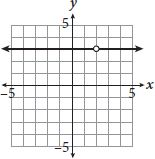Which of the following rational functions could represent the graph shown?

• A.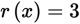• B.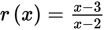• C.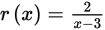• D.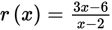Explanation:

D

Difficulty: Easy

Category: Passport to Advanced Math / Exponents

Strategic Advice: When matching a graph to an equation, look for key features of the graph, particularly when there is a feature that stands out.

Getting to the Answer: There is a "hole" in this graph (the open dot), which means that the function is not defined at a particular value of x, in this case x = 2 (the x-coordinate of the open dot). Think about what could cause that: A denominator that equals 0 when x = 2 is the correct response. Now look at the answer choices-you can eliminate A (because there is no denominator), and you can eliminate C (because the denominator is equal to 0 at x = 3, not x = 2). To choose between B and (D), simplify the functions to see which results in a horizontal line at r(x) = 3 (because the line is at y = 3). The function in B doesn't simplify at all, so skip it for now. For (D):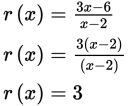The equation in (D) is indeed a horizontal line at y = 3 with a "hole" at x = 2.